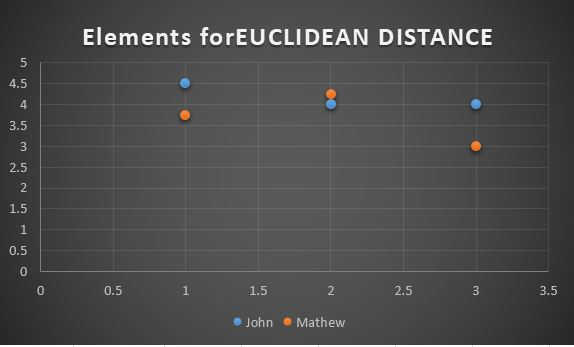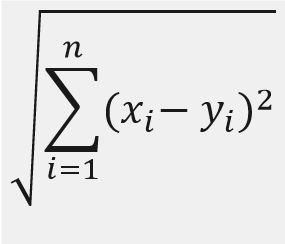New Delhi, India

# Euclidean Distance for finding Similarity# Euclidean Distance for finding Similarity

In this tutorial, we will learn how to use Euclidean distance for finding similarity. Have you ever thought that how we can judge whether the two people are similar or not, or in a group which two have highest similarity? If yes, then here is the answer. Let us understand it with an example, consider there is a group of four people and corresponding to each of them we have some data, in our case we have some ratings provided by them for some fruits, 5 means best and 0 means worst.

 Mango Banana Strawberry Pineapple Orange Apple John 4.5 3.5 4 4 Martha 2.5 4.5 5 3.5 Mathew 3.75 4.25 3 3.5 Nick 4 3 4.5 4.5

Now, if we want to compare John with Mathew, we can simply calculate the Euclidean Distance between the ratings they have provided corresponding to same item. Here Mango, Strawberry and Pineapple are the fruits which are common for both of them. Consider the rating corresponding each fruit be the distance elements. Lower the distance higher is the similarity.

### Euclidean Distance TheoryThis formula helps in calculating the Euclidean Distance, where ‘n’ is the total number of elements, ‘x’ and ‘y’ are the two distance elements.

In our example, total elements ‘n’ = 3

Value of ‘x’ corresponds to the ratings of fruits of John and value of ‘y’ corresponds to the ratings of fruits of Mathew. Euclidean distance for both of them is = 1.2747548783981961. Now, we need to normalize it, for that we can do the following

Result = (1 / (1 +Euclidean Distance))

For our example it comes out to be 0.439607805437114. ‘Result’ value always lies between 0 and 1, the value 1 corresponds to highest similarity.

### Python code for the above method

[sourcecode language=”python” wraplines=”false” collapse=”false”]
#Dictionary of People rating for fruits
choices={‘John’: {‘Mango’:4.5, ‘Banana’:3.5, ‘Strawberry’:4.0, ‘Pineapple’:4.0},
‘Nick’: {‘Mango’:4.0, ‘Orange’:4.5, ‘Banana’:3.0, ‘Pineapple’:4.5},
‘Martha’: {‘Orange’:5.0, ‘Banana’:2.5, ‘Strawberry’:4.5, ‘Apple’:3.5},
‘Mathew’: {‘Mango’:3.75, ‘Strawberry’:4.25, ‘Apple’:3.5, ‘Pineapple’:3.0}}

import pandas as pd

from math import sqrt

class testClass():
def create_csv(self):
df = pd.DataFrame.from_dict(choices, orient=’index’)
df.to_csv(‘fruits.csv’)

#Finding Similarity among people using Eucledian Distance Formula
def choice_distance(self, cho, per1, per2):
#Will set the following dictionary if data is common for two persons
sample_data={}
#Above mentioned varibale is an empty dictionary, that is length =0

for items in cho[per1]:
if items in cho[per2]:
sample_data[items]=1
#Value is being set 1 for those items which are same for both persons

#If both person does not have any similarity or similar items return 0
if len(sample_data)==0: return 0

#Calculating Euclidean Distance
final_sum = sum([pow(cho[per1][items]-cho[per2][items],2) for items in cho[per1] if items in cho[per2]])
return(1/(1+sqrt(final_sum)))
#Value being returned above always lies between 0 and 1
#Value 1 is added to sqrt to prevent 1/0 division and to normaloze result.

def main():
ob = testClass()
ob.create_csv()
print(ob.choice_distance(choices, ‘John’, ‘Nick’))
print(ob.choice_distance(choices, ‘John’, ‘Martha’))
print(ob.choice_distance(choices, ‘John’, ‘John’))

if __name__ == “__main__”:
main()
[/sourcecode]

### Output

0.5358983848622454
0.4721359549995794
1.0

There are many other mathematical models for calculating this type of similarity. In next article we will learn about Pearson Correlation Score, which is a bit complex way for finding out similarity among people.

Stay tuned and keep learning!! For more updates and news related to this blog as well as to data science, machine learning and data visualization, please follow our facebook page by clicking this link.

## 2 thoughts on “Euclidean Distance for finding Similarity”

This site uses Akismet to reduce spam. Learn how your comment data is processed.